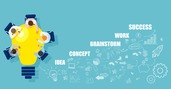Handling Categorical Variables with One-Hot Encoding

# Handling Categorical Variables with One-Hot Encoding

clickHere
Updated on Aug 9, 2023 15:21 IST

Handling categorical variables with one-hot encoding involves converting non-numeric categories into binary columns. Each category becomes a column with ‘1’ for the presence of that category and ‘0’ for others, enabling machine learning models to work with categorical data effectively while avoiding misinterpretation of ordinal relationships.

As a data science aspirant, you must have heard about one-hot encoding and ordinal encoding. And some of you must have faced the question that how you will handle the categorical variables in the interview also. This topic is very important from an interview point of view. So every aspiring data scientist needs to know the answer.

In this blog, you will learn about

• Categorical features.
• Dummy variables
• How to convert categorical features to numerical features by using one-hot encoding.

As we know Machines understand numbers, not text. So we need to convert each text category else the machine can’t process it. The same goes for machine learning algorithms. Ever wondered how we can do that? What are the different ways?

## What is Categorical Encoding?

Any dataset includes multiple columns – a combination of numerical as well as categorical variables. But as machines don’t understand categorical features/variables we need to convert categorical columns to numerical columns. This process is called categorical encoding.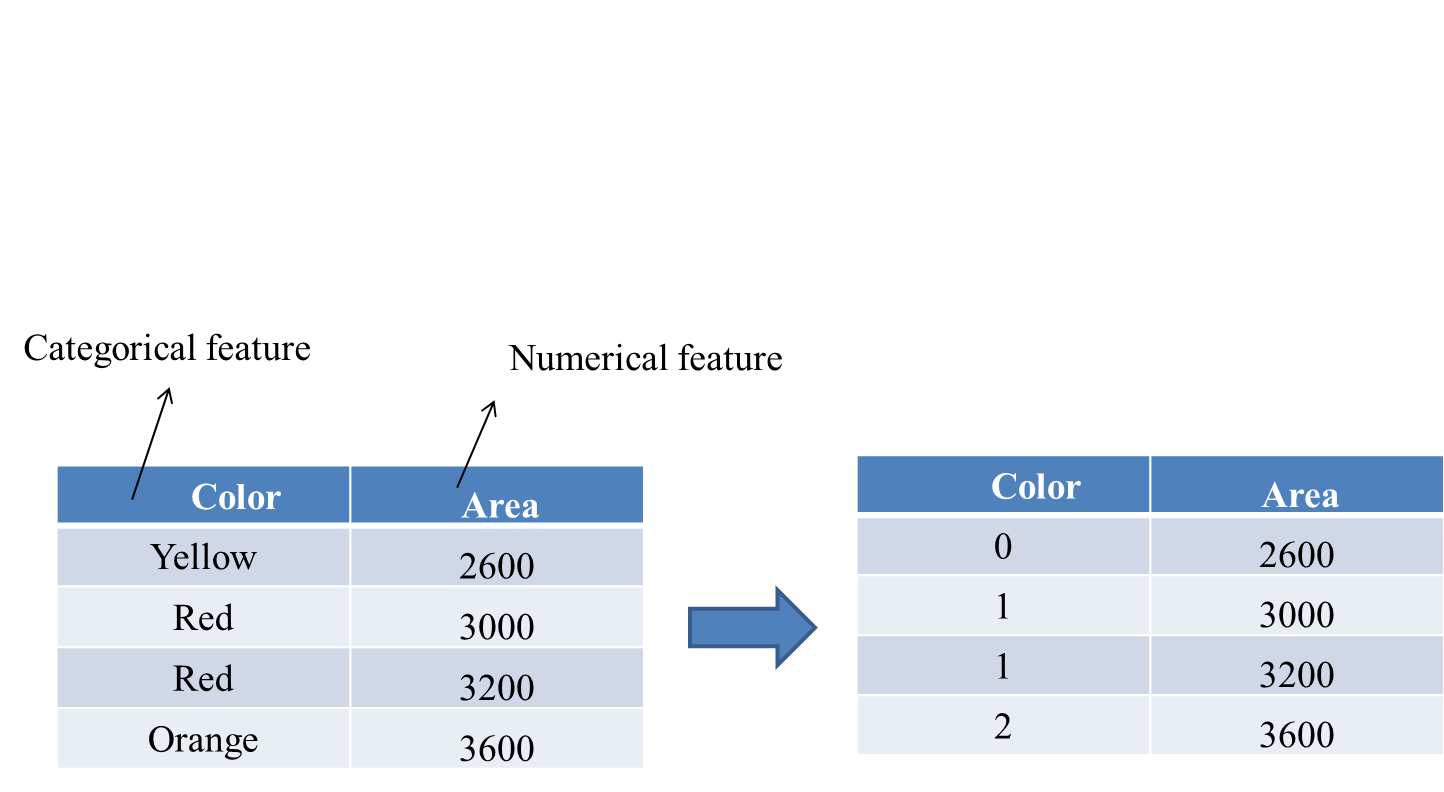Categorical Variables contain values that are names, labels, or strings. At first glance, these variables seem harmless. However, they can cause difficulties in your machine learning models as can be processed only when some numerical importance is given to them.

## Different Approaches to Categorical Encoding

So, how should we handle categorical variables? As it turns out, there are multiple ways of handling Categorical variables.

• Label Encoding
• One-Hot Encoding

In this blog, we will be focussing mostly on the one-Hot encoding technique.

### Ordinal Encoding

This technique is used for categorical variables where order matters.

Ordinal Encoding is a popular encoding technique for handling categorical variables. In this technique, each label is assigned a unique integer based on some ordering. Like in fig below we have a feature Education has three categories. Each category doesn’t have equal importance. As you know Ph.D. is the highest qualification so it is having a value of 1 and Graduation is the lowest qualification amongst them so it is having a value of 3

### One-Hot Encoding

One-Hot Encoding is the process of creating dummy variables. This technique is used for categorical variables where order does not matter.

One-Hot encoding technique is used when the features are nominal(do not have any order). In one hot encoding, for every categorical feature, a new variable is created. Categorical features are mapped with a binary variable containing either 0 or 1. Here, 0 represents the absence, and 1 represents the presence of that category.

These newly created binary features are known as Dummy variables. This is also known as Dummy encoding. Now the number of dummy variables depends on the number of categories present. This might sound a little complicated. But don’t worry I will explain this with an example.

Suppose we have a dataset with a category Country, which further has different countries like India, Australia, Russia, America. Now we have to one-hot encode this data.

So you can see that we have 4 categories i.e. India, Australia, Russia, and America. So we have 4 columns. And when we have India we got 1 as the value and the rest values are 0. And same applies to all categories.

We now have a new column for every class that exists in our Country column. Every category is represented in binary values, we have not assigned them any particular order or scale to the data. We have just created new columns(dummy variables) that the model can easily and fairly assess as to whether any predictive relationship exists.

We can now use these new columns as our input variables in our model and discard the Country column as the information contained is now completely represented by these 4 new numerical columns that the model can deal with. These new variables are dummy variables. And to do this, we will use the process that is called One-Hot Encoding.

## Dummy variable trap

The Dummy Variable Trap occurs when different  input variables perfectly predict each other – leading to multicollinearity.”

Multicollinearity is a scenario when two or more input variables are highly correlated with each other. This scenario we attempt to avoid as it won’t necessarily affect the overall predictive accuracy of the model.

Now, what’s the solution to the dummy variable trap? we drop one of the newly created columns produced by one-hot encoding. This can be done because the dummy variables include redundant information supposed, we have ‘n’ number of categories, we will drop one new created column(dummy variable) and can use ‘n−1’ dummy variables. This will prevent the dummy variable trap. Let’s understand the figure below.

You must be thinking that if we want to check for America. In that case, values for India, Australia, and Russia will be 0 all.

Let’s understand the Dummy variable with python code.

Also explore: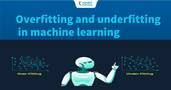Overfitting and Underfitting with a real-life example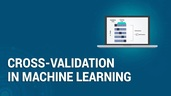Cross-validation techniques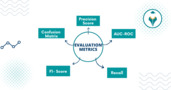Evaluation Metrics in Machine Learning
Evaluation metrics are the compass guiding machine learning models towards accuracy and efficiency. Dive into this article to unravel the significance of these metrics, from the classic AUC-ROC to the...read more

## One hot encoding Python

Suppose we want to predict the house price. In the dataset, we have the categorical variable Town, which we have to convert to a numerical variable. For this, we have used the home price.csv dataset.

### 1. Importing libraries and reading dataset

` `
`import pandas as pd      df = pd.read_csv("home price.csv")      dfCopy code`

### 2. Dummy variables

` `
`dummies = pd.get_dummies(df.town)  DummiesCopy code`

In this, we have three categories so had three columns for three different dummy variables.

### 3. Merging dummy variables

` `
`merged = pd.concat([df,dummies],axis=1)mergedCopy code`

### 4. Dropping ‘town’ column

` `
`final = merged.drop(['town'], axis=1)   finalCopy code`

As we have different dummy variables representing the same information as the town column. So we can drop the town column.

### 5. Dropping one dummy variable

` `
`final = final.drop(['robinsville'], axis=1)  finalCopy code`

Avoiding dummy variable trap we are dropping Robbinsville column here.

Note: You can drop anyone dummy variable column you want.

### 6. Independent variable

` `
`X = final.drop('price', axis=1)   XCopy code`

### 7. Dependent variable

` `
`y = final.priceCopy code`

### 8. Importing Linear Regression model

` `
`from sklearn.linear_model import LinearRegressionmodel = LinearRegression()Copy code`

### 9. Fitting the model

` `
`model.fit(X,y)Copy code`

### 10. Predicting the house price

` `
`model.predict(X)Copy code`

### 11. Checking the accuracy

` `
`model.score(X,y)Copy code`

Output:

0.9573929037221873

## Drawbacks of One-Hot and Dummy Encoding

One hot encoder and dummy encoder are very popular among the data scientists, But may not be as effective when-

• A large number of levels are present in the data.
• In the case of multiple categories in a feature variable, we need a similar number of dummy variables to encode the data. For example, a column with  25 different values will require 25 new variables for coding.
• The categorical features present in the data is ordinal(That means ordering matters in the categories)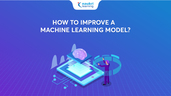How to improve machine learning model
After building machine learning the work is not over!! The next task is to improve machine learning model. But this is a challenging task. Some of you must have...read more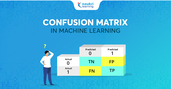Confusion Matrix in Machine Learning
Confusion Matrix is a simple technique for checking the performance of a classification model for a given set of test data.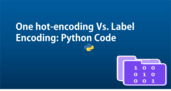One hot encoding vs label encoding in Machine Learning
As in the previous blog, we come to know that the machine learning model can’t process categorical variables. So when we have categorical variables in our dataset then we...read more

## Endnotes

Congrats on making it to the end!! You should have an idea of what one-hot encoding is and why it is used and how to use it. We have to first handle categorical variables before moving to other steps like training model, hyperparameter tuning, cross-validation, evaluating the model, etc.

Will be coming up with another blog related to how to handle label and ordinal features. Stay tuned!!!

If you liked my blog consider hitting the stars.

## FAQs

What are categorical variables?

Categorical variables are data attributes that represent different categories or labels, such as colors, types of objects, or any non-numeric values.

What is one-hot encoding?

One-hot encoding is a technique used to convert categorical variables into binary vectors. Each category becomes a new binary column, and for each observation, the corresponding column is marked with a '1' while others are marked with '0'.

Why use one-hot encoding?

One-hot encoding is used to transform categorical variables into a format that machine learning algorithms can understand. It prevents the algorithm from assuming any ordinal relationship between the categories.

How does one-hot encoding help in machine learning?

One-hot encoding prevents algorithms from treating categorical values as numbers, which can lead to incorrect assumptions. It ensures equal distance between categories and helps algorithms learn more effectively.

clickHere

This is a collection of insightful articles from domain experts in the fields of Cloud Computing, DevOps, AWS, Data Science, Machine Learning, AI, and Natural Language Processing. The range of topics caters to upski... Read Full Bio

## Trending Data Science CoursesMachine Learning
Coursera4.6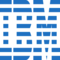Calculus for Machine Learning and Data Science
Coursera4.8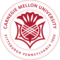## Top Picks & New Arrivals